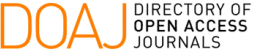### An Inverse Problem Approach for the Estimation of the Haverkamp and van Genuchten Retention Curves Parameters with the Luus-Jaakola Method

F. F. Ferreira, M. De O. Temperini, W. R. Telles, G. B. Lyra, A. J. Silva Neto

#### Abstract

In academia, the study of the movement of water in the ground has been widespread since the last century. The same can be determined using the Richards equation. This is a nonlinear parabolic partial differential equation that requires parameters to generate the results of the problem. Some authors have proposed equations that represent the relationship between volumetric moisture and soil water potential, such as Haverkamp and van Genuchten. As main objective of this work, the inverse modeling was implemented to obtain the Richards equation parameters, applying the Luus-Jaakola method. To verify the mathematical model, a sensitivity analysis was performed, which allowed the observation of the effect that each parameter has on the output data, implying a linear dependence. The results produced proved to be satisfactory for the problem analyzed in our research.

#### Keywords

Richards equation, Inverse problem, Luus-Jaakola

#### Full Text:

PDF

DOI: https://doi.org/10.5540/tcam.2021.022.02.00265

### Refbacks

• There are currently no refbacks.

Trends in Computational and Applied Mathematics

A publication of the Brazilian Society of Applied and Computational Mathematics (SBMAC)

Indexed in: